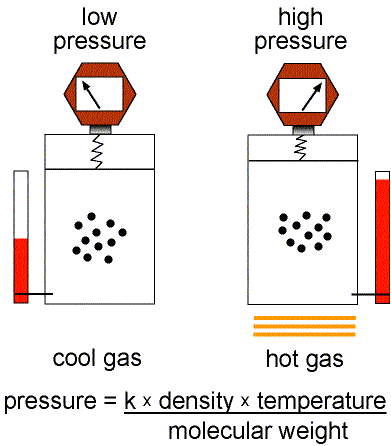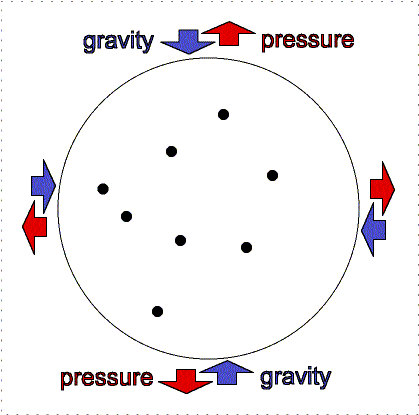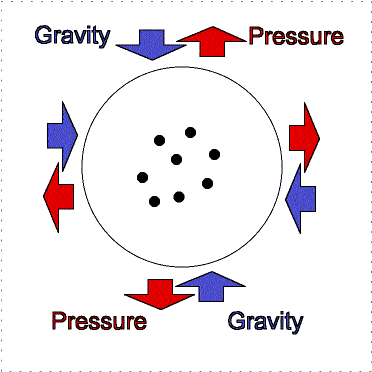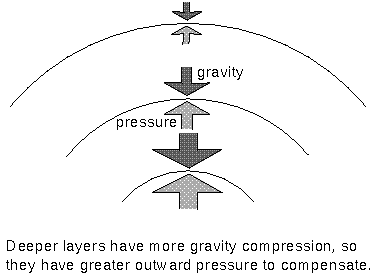## Interior Structure of Stars

#### Chapter index in this window —   — Chapter index in separate window

This material (including images) is copyrighted!. See my copyright notice for fair use practices.

Observations of the stars in all regions of the electromagnetic spectrum and careful observations of the Sun's pulsation modes and neutrinos provide the data needed to construct models of the interiors of stars. This section is about how to find out what the interior of a star is like without physically taking one apart (a rather difficult thing to do).

### Mathematical Models

Astronomers construct mathematical models of the interior of a star using the information pouring from the surfaces of stars (especially the Sun) and their knowledge of how gases behave under different conditions. The mathematical models are a set of equations that describe how things work layer by layer in a star. Fortunately, the interior of stars is completely gaseous all the way to the center, so the equations are relatively simple (whew!). The physics of gases can be described with just three parameters:
1. Temperature---a measure of the random motion energy (the average kinetic energy) of the gas particles. The higher the temperature, the more random kinetic energy is present.

2. Pressure---the amount of force/area. Hot gas expands to create pressure on its surroundings. For example, the gas inside a hot air balloon pushes out on the material of the balloon enclosing the gas.

3. Mass Density---the amount of mass/volume. Gaseous material can be compressed to smaller volumes and higher densities.

### Equation of State

How the three parameters work together to describe the material you are studying is determined by the equation of state of the material. This is an equation that relates density, pressure, and temperature. The equation of state for solids and liquids is very complex and uncertain. The equation of state for the gas is simple: the pressure = (a constant × the mass density × the temperature) / (the molecular weight of the gas). The molecular weight of a particular type of gas is the combined mass of all of the isotopes of that type of gas in the proportions found in nature. For hydrogen, the molecular weight is very close to 1; for helium, the molecular weight is very close to 4. For a gas made of different types of atoms (such as that found in stars), the molecular weight is the weighted mean of the different atomic types, taking into account the relative proportions of the different types of atoms. This equation of state for simple gases is also called the ideal gas law.### Gravity Holds a Star Together

Stars are held together by gravity. Gravity tries to compress everything to the center. What holds an ordinary star up and prevents total collapse is thermal and radiation pressure. The thermal and radiation pressure tries to expand the star layers outward to infinity.Hydrostatic equilibrium: gravity compression is balanced by pressure outward.Greater gravity compresses the gas, making it denser and hotter, so the outward pressure increases.

In any given layer of a star, there is a balance between the thermal pressure (outward) and the weight of the material above pressing downward (inward). This balance is called hydrostatic equilibrium. A star is like a balloon. In a balloon the gas inside the balloon pushes outward and the elastic material supplies just enough inward compression to balance the gas pressure. In a star the star's internal gravity supplies the inward compression. Gravity compresses the star into the most compact shape possible: a sphere. Stars are round because gravity attracts everything in an object to the center. Hydrostatic equilibrium also explains why the Earth's atmosphere does not collapse to a very thin layer on the ground and how the tires on your car or bicycle are able to support the weight of your vehicle.Long before astronomers knew about nuclear fusion, they had a good idea of how the density and temperature of stars increased toward their cores. Deeper layers have more gravity compression from the overlying layers. The greater gravity compression raises the density of the gas. In order to balance the greater gravity compression, the outward pressure of the gas and radiation is increased by raising the temperature. Calculating the change in density and temperature layer by layer toward the center of a star, you find the temperature at the core of a star = 8 to 28 million K and the densities = 10 to 130 times the density of water. As stars age, these numbers increase! You have already seen in the previous section that hydrostatic equilibrium also provides a ``thermostatic control'' on the energy generation inside a star and keeps the star stable.

### Other Pieces

Other basic physical principles are put into the mathematical models:
1. Continuity of Mass: the total stellar mass = sum of all of the shell layer masses. Mass is distributed smoothly throughout star's interior (there are no gaps or pockets of ``negative'' mass). Also, the law of the conservation of mass says that the total amount of mass does not change with time.

2. Continuity of Energy: the amount of energy flowing out the top of each shell layer in a star = the amount of energy flowing in at bottom of the shell layer. No energy is magically destroyed or created from nothing. A star's luminosity = sum of all of the shell layer energies. Also, the law of the conservation of energy says that the total amount of energy does not change with time. Energy can change from one form to another form of energy, but the total amount is a constant.

3. Energy Transport: Recall from the discussion about how energy flows in planetary atmospheres that energy moves from hot to cold via conduction, radiation, or convection. Nature will first try to use radiation (photons) to move energy from the very hot interior to the very cold space. If radiation cannot transport all of the energy over the distance from the center to the surface of the star, then nature will also use convection. Convection is the bulk motion of gases used to transport energy. Hot gases rise to the upper levels and radiate their extra energy at the upper levels while cooler gases sink to pick up more energy from the hot interior. Conduction transports energy by having each atom transfer its energy to the atom next to it. Conduction is not an efficient process in a gas so it transports a very small amount of energy in stars and is usually ignored.

4. Opacity: It takes a LONG time for photons produced by nuclear reactions in the core to reach the surface. In the opaque interior a photon travels only about 1 centimeter before it runs into an atom or ion and is absorbed. A measure of the gas' ability to absorb the photons is called its opacity. You cannot see into the interior of a star because the gas has a high opacity.

The photon is later re-emitted but in a random direction. It may be re-emitted in the direction it came from! So the photon travels a very zig-zag sort of path outward. It takes about a million years for a photon to travel from where it was created in the core to the surface where it is finally released into space. Along the way the photon has transferred some its energy to the gas particles, so the photon has changed from very high energy gamma rays to the lower energy visible light photons. Some of the radiation is also in the form of neutrinos. The gas has almost zero opacity with the neutrinos so they pass right on through the star's gas in just a few seconds.

5. The equation of state, hydrostatic equilibrium and the other physical principles are put together for each layer in a star. The equations are solved for each layer starting from the layer there is direct information of, the surface. That result gives the conditions for the next layer's equations. Solving the layer's equations gives the conditions for the layer below it and this process continues on down toward the center layer by layer. In order to get sufficient detail for accurate results, the star's interior is divided into hundreds of layers. To save on time, the equations are solved using a computer.

#### Go to Astronomy Notes home

last updated: June 6, 2019

##### Is this page a copy of Strobel's Astronomy Notes?

Author of original content: Nick Strobel